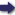(London :  Kegan Paul, Trench, Trübner & Co.,  1910.)

 Tools

## Search this bookPrev Page 189 Next``` CHAPTER LXXVII. 189 count off the week-days, beginning with the beginning of Sunday. That time you arrive at is the moment of sctmkrdnti. What must he added to the Basis. The Zodiacal Signs. Days. 3 (iliaiT. Cashaka. Aries i 19 0 Taurus 6 17 , 0 Gemini 2 43 0 Cancer 6 21 0 Leo . 2 49 0 Virgo 5 49 0 Libra I 14 0 Scorpio 3 6 30 Arcitenens 4 34 30 Capricornus 5 54 0 Amphora . 0 30 0 Pisces 2 JI 20 The beginning of consecutive solar years in the week On the differs by I day and the fraction at the end of the tiie"soiar year. This amount, reduced to fractions of one kind, ing to Brah- is the multiplicator (180), used in the preceding com- Pu&a, kiid putation in order to find the surplus of each year (i.e. * ''^'^ ^^'"^' the amount by which its beginning wanders onward through the week). The divisor (143) is the denominator of the fraction (which is accordingly -rff)- Accordingly the fraction at the end of the solar year is, in this computation, reckoned as {^'^, which implies as the length of the solar year, 365 days 15^31'^ 28''' 6"'. To raise this fraction of a day to one whole day, iff of a day are required. I do not know whose theory this is. If we divide the days of a cctturyugct by the number of its solar years, according to the theory of Brahma¬ gupta, we get as the length of the solar year, 365 days 30 22'^ 30''' 0'\ In this case the multiplicator or gunakdrct is 4027, and the divisor or bhdgcthdra is 3200 Page 293. (i.e. I day 30' 22" 30''^ o''' are equal to if-g-g-). ```Prev Page 189 Next